• python专家系统
千次阅读
2021-06-19 12:20:54

只给两个课时的人工智能作业动物专家系统实验，局限性很多。
设置规则时1-8时已经最大可能避免的规则冲突，所以基本没有规则冲突处理。
对规则9-15采用FIRST法处理冲突。
PS：前端界面如果有时间会做的，到时候更新贴出来。
一 实验目的
熟悉掌握基于规则系统的表示与推理
二 实验内容
设计一个动物识别专家系统，规则库至少包含15条规则，可以识别至少7种动物，规则可增加；
界面显示要求:
1）有若干选择动物特征的选择列表；
2）表现判断动物时，使用了哪些规则;
3）表现数据库的变化；
4）显示规则的调用次序；
5）显示最后的结果，包含动物能识别出来和动物不能识别出来两种情况；
6）至少检查两个例子实现正向推理和反向推理的区别
三 设计思路
1.设计综合数据库

features = ["有奶", "有毛发", "有羽毛", "会飞", "会下蛋",  \
"吃肉", "有犬齿", "有爪","眼盯前方", "有蹄", "嚼反刍",\
"黄褐色", "身上有暗斑点", "身上有黑色条纹", "有长脖子", \
"有长腿", "不会飞", "会游泳", "有黑白二色", "善飞",\
"哺乳动物", "鸟", "食肉动物", "蹄类动物", "金钱豹", "虎",\
"长颈鹿", "斑马", "鸵鸟", "企鹅", "信天翁"]


2.设计规则库

rule1 =    # if 动物有毛发2  then  动物是哺乳动物21
rule2 =    # if 动物有奶1  then  动物是哺乳动物21

rule3 =    # if 动物有羽毛3  then  动物是鸟22
rule4 = [4, 5]  # if 动物会飞4 and  会生蛋5 then  动物是鸟22

rule5 =   # if 动物吃肉6 then 动物是食肉动物23
rule6 = [7, 8, 9]  # if 动物有犀利牙齿7 and 有爪8 and 眼向前方9 then 动物是食肉动物23

rule7 = [21, 10]  # if 动物是哺乳动物21 and 有蹄10 then 动物是有蹄类动物24
rule8 = [21, 11]  # if 动物是哺乳动物21 and 反刍11 then 动物是有蹄类动物24

rule9 = [21, 23, 12, 13]  # if 动物是哺乳动物21 and 是食肉动物23 and 有黄褐色12 and 有暗斑点13 then 动物是豹25
rule10 = [21, 23, 12, 14]  # if 动物是哺乳动物21 and 是食肉动物23 and 有黄褐色12 and 有黑色条纹14 then 动物是虎26
rule11 = [24, 15, 16, 13]  # if动物是有蹄类动物24  and 有长脖子15 and 有长腿16 and 有暗斑点13 then 动物是长颈鹿27
rule12 = [24, 14]  # if 动物是有蹄类动物24 and 有黑色条纹14 then 动物是斑马28
rule13 = [22, 17, 16, 15, 19]  # if 动物是鸟22 and 不会飞17 and 有长脖子16 and 有长腿15 and 有黑白二色15 then 动物是鸵鸟29
rule14 = [22, 17, 18, 19]  # if 动物是鸟22 and 不会飞17 and 会游泳18 and 有黑白二色19 then  动物是企鹅30
rule15 = [22, 4, 5]  # if 动物是鸟22 and善飞4 and 会生蛋5 then 动物是信天翁31


其中rule1-rule8为动物特征推导动物特征的规则，rule9-rule15为动物特征推导动物种类的规则
3.推理原理
正向推理：
a.input手动选择特征生成事实数据库，根据这些特征推导出其他动物特征，最终推导出动物种类
b.判断输入的事实库是否包含规则1-8的集合，如果包含则向事实数据库中加入相应规则对应的事实。
如：选择特征中包含特征2则可根据rule1: if 动物有毛发2 then 动物是哺乳动物21，向事实数据库中加入特征21
c.判断输入的事实数据库是否包含规则9-15的集合，如果包含则输出相应规则所对应的动物
如：经过上述规则1-8推导后的事实数据库为【4,5,21,22】，rule15 = [22, 4, 5]为其子集，则可判定动物为信天翁31

answer = input('\n请选择动物的特征编号，用空格隔开，回车结束输入：')

print("正向推理过程如下：")
print('rule1：2->21\tif 动物有毛发2  then  动物是哺乳动物21')
print('rule2：1->21\tif 动物有奶1  then  动物是哺乳动物21')
print('rule3：3->22\tif 动物有羽毛3  then  动物是鸟22')
print('rule4：4+5->22\tif 动物会飞4 and  会生蛋5 then  动物是鸟22')
print('rule5：6->23\tif 动物吃肉6 then 动物是食肉动物23')
print('rule6：7+8+9->23\tif 动物有犀利牙齿7 and 有爪8 and 眼向前方9 then 动物是食肉动物23')
print('rule7：21+10->24\tif 动物是哺乳动物21 and 有蹄10 then 动物是有蹄类动物24')
print('rule8：21+11->24\tif 动物是哺乳动物21 and 反刍11 then 动物是有蹄类动物24')

print("if 动物是哺乳动物21 and 是食肉动物23 and 有黄褐色12 and 有暗斑点13 then 动物是豹25")
print("结果为：", end=" ")
print(features)
print("if 动物是哺乳动物21 and 是食肉动物23 and 有黄褐色12 and 有黑色条纹14 then 动物是虎26")
print("结果为：", end=" ")
print(features)
print("if动物是有蹄类动物24  and 有长脖子15 and 有长腿16 and 有暗斑点13 then 动物是长颈鹿27")
print("结果为：", end=" ")
print(features)
print("if 动物是有蹄类动物24 and 有黑色条纹14 then 动物是斑马28")
print("结果为：", end=" ")
print(features)
print("if 动物是鸟22 and 不会飞17 and 有长脖子16 and 有长腿15 and 有黑白二色15 then 动物是鸵鸟29")
print("结果为：", end=" ")
print(features)
print("if 动物是鸟22 and 不会飞17 and 会游泳18 and 有黑白二色19 then  动物是企鹅30")
print("结果为：", end=" ")
print(features)
print("if 动物是鸟22 and善飞4 and 会生蛋5 then 动物是信天翁31")
print("结果为：", end=" ")
print(features)


逆向推理：
A.输入事实数据库，和目标动物
B.根据规则9-15将对应动物所需的事实导出
如，选择动物金钱豹25，则所需事实为：[21, 23, 12, 13]
C.循环遍历需要的事实列表，如**[21, 23, 12, 13]**。判断每一个元素是否在事实数据库中存在，如果存在则继续遍历，不存在则判断事实库中中是否包含能够推出此事实的规则，如：21可根据规则1、2推导出如果有则继续遍历，没有，则推理失败。
遍历所需事实列表结束且每个事实都能存在于事实数据库，则推理成功

    animal=int(input('\n请选择动物的种类编号，回车结束输入：'))
# 接收到ans的wer是一个字符串
print("逆向推理过程如下：")
real=[]
if animal==25:
print(features[animal-1],'rule9：25->21,23,12,13  if 动物是哺乳动物21 and 是食肉动物23 and 有黄褐色12 and 有暗斑点13 then 动物是豹25')
real=rule9
elif animal==26:
print(features[animal-1],'rule10：26->21,23,12,114  if 动物是哺乳动物21 and 是食肉动物23 and 有黄褐色12 and 有黑色条纹14 then 动物是虎26')
real=rule10
elif animal==27:
print(features[animal-1],'rule11：27->24, 15, 16, 13  if动物是有蹄类动物24  and 有长脖子15 and 有长腿16 and 有暗斑点13 then 动物是长颈鹿27')
real=rule11
elif animal==28:
print(features[animal-1],'rule12：28->24, 14  if 动物是有蹄类动物24 and 有黑色条纹14 then 动物是斑马28')
real=rule12
elif animal==29:
print(features[animal-1],'rule13：29->22, 17, 16, 15, 19  if 动物是鸟22 and 不会飞17 and 有长脖子16 and 有长腿15 and 有黑白二色15 then 动物是鸵鸟29')
real=rule13
elif animal==30:
print(features[animal-1],'rule14：30->22, 17, 18, 19  if 动物是鸟22 and 不会飞17 and 会游泳18 and 有黑白二色19 then  动物是企鹅30')
real=rule14
elif animal==31:
print(features[animal-1],'rule15：31->22, 4, 5  if 动物是鸟22 and善飞4 and 会生蛋5 then 动物是信天翁31')
real=rule15
key=0
for i in real:
print(i)
continue
elif i ==21:
print("rule1:21->2  if 动物有毛发2  then  动物是哺乳动物21")
continue
print("rule2:21->1  if 动物有奶1  then  动物是哺乳动物21")
continue
elif i==22:
print("rule3:22->3  if 动物有羽毛3  then  动物是鸟22")
continue
print("rule4:22->4,5  if 动物会飞4 and  会生蛋5 then  动物是鸟22")
continue
elif i == 23:
print("rule5:23->6  if 动物吃肉6 then 动物是食肉动物23")
continue
print("rule6:23->7,8,9  if 动物有犀利牙齿7 and 有爪8 and 眼向前方9 then 动物是食肉动物23")
continue
elif i == 24:
print("rule7:24->21,10  if 动物是哺乳动物21 and 有蹄10 then 动物是有蹄类动物24")
continue
print("rule8:24->21,11  if 动物是哺乳动物21 and 反刍11 then 动物是有蹄类动物24")
continue
else:
key=1
if key==0:
print("推导成功！")
elif key==1:
print("推导失败！")


四 完整代码

#特征，综合数据库
features = ["有奶", "有毛发", "有羽毛", "会飞", "会下蛋",  \
"吃肉", "有犬齿", "有爪","眼盯前方", "有蹄", "嚼反刍",\
"黄褐色", "身上有暗斑点", "身上有黑色条纹", "有长脖子", \
"有长腿", "不会飞", "会游泳", "有黑白二色", "善飞",\
"哺乳动物", "鸟", "食肉动物", "蹄类动物", "金钱豹", "虎",\
"长颈鹿", "斑马", "鸵鸟", "企鹅", "信天翁"]

#规则库
rule1 =    # if 动物有毛发2  then  动物是哺乳动物21
rule2 =    # if 动物有奶1  then  动物是哺乳动物21

rule3 =    # if 动物有羽毛3  then  动物是鸟22
rule4 = [4, 5]  # if 动物会飞4 and  会生蛋5 then  动物是鸟22

rule5 =   # if 动物吃肉6 then 动物是食肉动物23
rule6 = [7, 8, 9]  # if 动物有犀利牙齿7 and 有爪8 and 眼向前方9 then 动物是食肉动物23

rule7 = [21, 10]  # if 动物是哺乳动物21 and 有蹄10 then 动物是有蹄类动物24
rule8 = [21, 11]  # if 动物是哺乳动物21 and 反刍11 then 动物是有蹄类动物24

rule9 = [21, 23, 12, 13]  # if 动物是哺乳动物21 and 是食肉动物23 and 有黄褐色12 and 有暗斑点13 then 动物是豹25
rule10 = [21, 23, 12, 14]  # if 动物是哺乳动物21 and 是食肉动物23 and 有黄褐色12 and 有黑色条纹14 then 动物是虎26
rule11 = [24, 15, 16, 13]  # if动物是有蹄类动物24  and 有长脖子15 and 有长腿16 and 有暗斑点13 then 动物是长颈鹿27
rule12 = [24, 14]  # if 动物是有蹄类动物24 and 有黑色条纹14 then 动物是斑马28
rule13 = [22, 17, 16, 15, 19]  # if 动物是鸟22 and 不会飞17 and 有长脖子16 and 有长腿15 and 有黑白二色15 then 动物是鸵鸟29
rule14 = [22, 17, 18, 19]  # if 动物是鸟22 and 不会飞17 and 会游泳18 and 有黑白二色19 then  动物是企鹅30
rule15 = [22, 4, 5]  # if 动物是鸟22 and善飞4 and 会生蛋5 then 动物是信天翁31

print('以下是一些动物的特征：')
i = 0
while i < 24:
print('%d' %(i+1) +'.'+ features[i]+ '  ', end='')
i = i+1
if i % 8 == 0:
print('\n')

print('以下是可识别的动物：')
while i < 31:
print('%d' %(i+1) +'.'+ features[i]+ '  ', end='')
i = i+1

flag= int(input('\n请选择\n1:正向推理\n2:反向推理\n'))
if flag==1:

print("正向推理过程如下：")
print('rule1：2->21\tif 动物有毛发2  then  动物是哺乳动物21')
print('rule2：1->21\tif 动物有奶1  then  动物是哺乳动物21')
print('rule3：3->22\tif 动物有羽毛3  then  动物是鸟22')
print('rule4：4+5->22\tif 动物会飞4 and  会生蛋5 then  动物是鸟22')
print('rule5：6->23\tif 动物吃肉6 then 动物是食肉动物23')
print('rule6：7+8+9->23\tif 动物有犀利牙齿7 and 有爪8 and 眼向前方9 then 动物是食肉动物23')
print('rule7：21+10->24\tif 动物是哺乳动物21 and 有蹄10 then 动物是有蹄类动物24')
print('rule8：21+11->24\tif 动物是哺乳动物21 and 反刍11 then 动物是有蹄类动物24')

print("if 动物是哺乳动物21 and 是食肉动物23 and 有黄褐色12 and 有暗斑点13 then 动物是豹25")
print("结果为：", end=" ")
print(features)
print("if 动物是哺乳动物21 and 是食肉动物23 and 有黄褐色12 and 有黑色条纹14 then 动物是虎26")
print("结果为：", end=" ")
print(features)
print("if动物是有蹄类动物24  and 有长脖子15 and 有长腿16 and 有暗斑点13 then 动物是长颈鹿27")
print("结果为：", end=" ")
print(features)
print("if 动物是有蹄类动物24 and 有黑色条纹14 then 动物是斑马28")
print("结果为：", end=" ")
print(features)
print("if 动物是鸟22 and 不会飞17 and 有长脖子16 and 有长腿15 and 有黑白二色15 then 动物是鸵鸟29")
print("结果为：", end=" ")
print(features)
print("if 动物是鸟22 and 不会飞17 and 会游泳18 and 有黑白二色19 then  动物是企鹅30")
print("结果为：", end=" ")
print(features)
print("if 动物是鸟22 and善飞4 and 会生蛋5 then 动物是信天翁31")
print("结果为：", end=" ")
print(features)
else:
print('识别失败！')
elif flag==2:
animal=int(input('\n请选择动物的种类编号，回车结束输入：'))
# 接收到ans的wer是一个字符串
print("逆向推理过程如下：")
real=[]
if animal==25:
print(features[animal-1],'rule9：25->21,23,12,13  if 动物是哺乳动物21 and 是食肉动物23 and 有黄褐色12 and 有暗斑点13 then 动物是豹25')
real=rule9
elif animal==26:
print(features[animal-1],'rule10：26->21,23,12,114  if 动物是哺乳动物21 and 是食肉动物23 and 有黄褐色12 and 有黑色条纹14 then 动物是虎26')
real=rule10
elif animal==27:
print(features[animal-1],'rule11：27->24, 15, 16, 13  if动物是有蹄类动物24  and 有长脖子15 and 有长腿16 and 有暗斑点13 then 动物是长颈鹿27')
real=rule11
elif animal==28:
print(features[animal-1],'rule12：28->24, 14  if 动物是有蹄类动物24 and 有黑色条纹14 then 动物是斑马28')
real=rule12
elif animal==29:
print(features[animal-1],'rule13：29->22, 17, 16, 15, 19  if 动物是鸟22 and 不会飞17 and 有长脖子16 and 有长腿15 and 有黑白二色15 then 动物是鸵鸟29')
real=rule13
elif animal==30:
print(features[animal-1],'rule14：30->22, 17, 18, 19  if 动物是鸟22 and 不会飞17 and 会游泳18 and 有黑白二色19 then  动物是企鹅30')
real=rule14
elif animal==31:
print(features[animal-1],'rule15：31->22, 4, 5  if 动物是鸟22 and善飞4 and 会生蛋5 then 动物是信天翁31')
real=rule15
key=0
for i in real:
print(i)
continue
elif i ==21:
print("rule1:21->2  if 动物有毛发2  then  动物是哺乳动物21")
continue
print("rule2:21->1  if 动物有奶1  then  动物是哺乳动物21")
continue
elif i==22:
print("rule3:22->3  if 动物有羽毛3  then  动物是鸟22")
continue
print("rule4:22->4,5  if 动物会飞4 and  会生蛋5 then  动物是鸟22")
continue
elif i == 23:
print("rule5:23->6  if 动物吃肉6 then 动物是食肉动物23")
continue
print("rule6:23->7,8,9  if 动物有犀利牙齿7 and 有爪8 and 眼向前方9 then 动物是食肉动物23")
continue
elif i == 24:
print("rule7:24->21,10  if 动物是哺乳动物21 and 有蹄10 then 动物是有蹄类动物24")
continue
print("rule8:24->21,11  if 动物是哺乳动物21 and 反刍11 then 动物是有蹄类动物24")
continue
else:
key=1
if key==0:
print("推导成功！")
elif key==1:
print("推导失败！")人工智能 算法
更多相关内容
• 考察 产生式系统 我知道在搜索引擎的茫茫大海中，你能看见我的这篇文章不是缘分，而是你要交作业了 拿代码去“学习”之前，至少点个赞吧 代码没写GUI，因为不喜欢这玩意，直接在终端中进行人机交互。使用代码之前，...
• 专业系统 基于 Python专家系统回答问题服务器上的未决问题在本地环境中安装先决条件： Python 点子virtualenv (pip install --user virtualenv) 命令行界面转到您的本地工作区 cd /path/to/your/workspace/从 ...
• 一个简单的专家系统，PYQT5做的界面，有兴趣的可以看看
• 1.利用pyqt5来设计专家系统的界面。如下图一：图一 起始界面相应的运行过程的界面，如图二：图二 相应的运行过程界面2.设置规则文本 guizhe.txt有毛发 哺乳动物有奶 哺乳动物有羽毛 鸟会飞 下蛋 鸟吃肉 食肉动物有...

1. 利用pyqt5来设计专家系统的界面。

如下图一：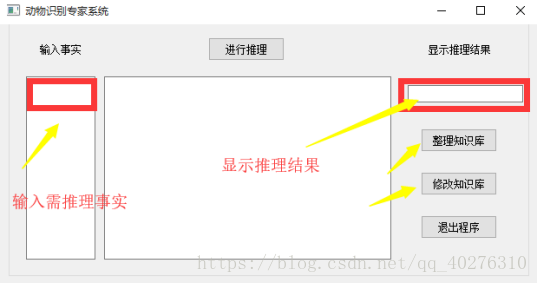图一 起始界面

相应的运行过程的界面，如图二：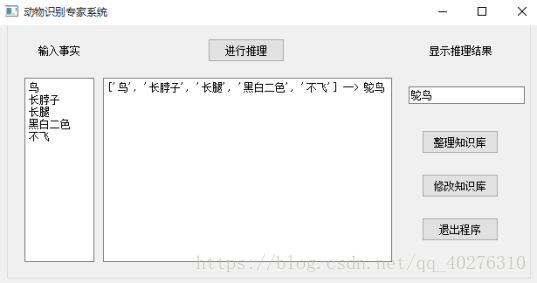图二 相应的运行过程界面

2. 设置规则文本 guizhe.txt

有毛发 哺乳动物

有奶 哺乳动物

有羽毛 鸟

会飞 下蛋 鸟

吃肉 食肉动物

有犬齿 有爪 眼盯前方 食肉动物

哺乳动物 有蹄 有蹄类动物

哺乳动物 嚼反刍动物 有蹄类动物

哺乳动物 食肉动物 黄褐色 暗斑点 金钱豹

哺乳动物 食肉动物 黄褐色 黑色条纹 虎

有蹄类动物 长脖子 长腿 暗斑点 长颈鹿

有蹄类动物 黑色条纹 斑马

鸟 长脖子 长腿 黑白二色 不飞 鸵鸟

鸟 会游泳 不飞 黑白二色 企鹅

鸟 善飞 信天翁

3. 编写思路

1)首先将规则库里面的规则进行相应的排序和数据处理，以便之后的推理信息的匹配，在这里我们将规则信息进行相应的拓扑排序，代码如下：

inn=[]foriinP:sum=0forxini:ifQ.count(x)>0:# 能找到，那么sum+=Q.count(x)inn.append(sum)while(1):x=0ifinn.count(-1)==inn.__len__():breakforiininn:ifi==0:str=' '.join(P[x])# print("%s %s" %(str, Q[x]))ans=ans+str+" "+Q[x]+"\n"# 写入结果# print("%s -- %s" %(P[x],Q[x]))inn[x]= -1# 更新入度y=0forjinP:ifj.count(Q[x])==1:inn[y]-=1y+=1x+=1

2)整理好规则库之后，我们开始进行推理功能的编写，在这里我们根据相应的规则匹配模式，对用户输入的需要推理的信息进行相应的判断，然后一步步的询问用户相关的规则信息，进行进一步的推理，直到完全匹配出推理信息。代码如下：

defgo(self,flag=True):self.Q=[]self.P=[]fo=open('guizhe.txt','r',encoding='utf-8')forlineinfo:line=line.strip('\n')ifline=='':continueline=line.split(' ')self.Q.append(line[line.__len__()-1])del(line[line.__len__()-1])self.P.append(line)fo.close()print("go按钮按下")self.lines=self.textEdit.toPlainText()self.lines=self.lines.split('\n')# 分割成组self.DB=set(self.lines)print(self.DB)self.str=""print(self.str)flag= Truetemp=""forxinself.P:# 对于每条产生式规则ifListInSet(x,self.DB):# 如果所有前提条件都在规则库中self.DB.add(self.Q[self.P.index(x)])temp=self.Q[self.P.index(x)]flag= False# 至少能推出一个结论# print("%s --> %s" %(x, self.Q[self.P.index(x)]))self.str+="%s --> %s\n"%(x,self.Q[self.P.index(x)])ifflag:# 一个结论都推不出print("一个结论都推不出")forxinself.P:# 对于每条产生式ifListOneInSet(x,self.DB):# 事实是否满足部分前提flag1= False# 默认提问时否认前提foriinx:# 对于前提中所有元素ifinot inself.DB:# 对于不满足的那部分btn=s.quest("是否"+i)ifbtn==QtWidgets.QMessageBox.Ok:self.textEdit.setText(self.textEdit.toPlainText()+"\n"+i)# 确定则增加到textEditself.DB.add(i)# 确定则增加到规则库中flag1= True# 肯定前提# self.go(self)ifflag1:# 如果肯定前提，则重新推导self.go()returnself.textEdit_2.setPlainText(self.str)print("----------------------")print(self.str)ifflag:btn=s.alert("啥也推不出来!!!")# if btn == QtWidgets.QMessageBox.Ok:  # 点击确定#     self.textEdit.setText(self.textEdit.toPlainText() + "\n确定")else:self.lineEdit.setText(temp)

3)询问用户补充的推理信息进行进一步推理，代码如下：

defquest(self,info):QtWidgets.QMessageBox.move(self,200,200)button=QtWidgets.QMessageBox.question(self,"Question",self.tr(info),QtWidgets.QMessageBox.Ok|QtWidgets.QMessageBox.Cancel,QtWidgets.QMessageBox.Cancel)returnbutton

4. 代码的优化处理

在编写的过程中，我们参考了相关的文献资料，思考是否可以通过更新规则库来扩展系统可识别的动物的数量，从而使得系统更加完善。于是在原基础上，通过参考大牛的代码，增添了“修改规则库”和“整理规则库”两个功能。代码如下：

classSecondWindow(QtWidgets.QWidget):def__init__(self,parent=None):super(SecondWindow,self).__init__(parent)self.setWindowTitle("修改规则库")self.setGeometry(725,200,300,300)self.textEdit=QtWidgets.QTextEdit(self)self.textEdit.setGeometry(8,2,284,286)

实验过程中遇到的问题如何解决的？

1. 遇到的问题

在本次实验中最大的问题就是第一步对于规则库的信息的整理和之后如何在匹配这些规则，开始使用了元组和集合，但是都难以进行多次匹配，于是想到了Python本身的字典的“键值对”属性，并且参考了相关文献，对信息做了拓扑排序。

2. 如何解决

首先，对规则信息通过字典的格式存入相应的“guizhe.txt”文件中，然后对当中的信息进行相应的拓扑排序。

本次实验的体会(结论)

1. 结论

本次实验项目，通过pyqt5与Eric6自动生成用户交互页面，体现了Python在前台的优势，同时整体的代码量比起用C/C++而言，大大减少，体现了Python在编写专家系统的优势。

2. 体会与项目优化

在后期查看相应查看大量有关专家系统的文件中，个人认为可以结合知识图谱来构建一个大型的动物专家系统的识别，我们可以通过复旦大学的知识工厂的相应的知识库构建相应的知识图谱，结合MongoDB数据库以及知识图谱的数据库，来构建一个更智能更全面的的动物识别的专家系统。当然所花的时间和精力也是非常巨大的！

展开全文• 自己用python写的玩具分类专家系统，自己写的，很简单能运行，欢迎大家互相交流！
• 动物识别专家系统（源码+简单界面+实验报告） （1）题目事实（概念） char *feature[]={"有毛","产奶","有羽毛","会飞","会下蛋","吃肉","有犬齿","有爪","眼睛盯前方","有蹄","反刍","黄褐色","有斑点","有黑色条纹...
• 其实一直对电影里面的对×××进行人脸匹配然后，刷出来×××信息很感兴趣，今天晚上一时兴起，就搞了一把小的。理论上：你可以建立一个你感兴趣的百万级的数据库，给你个照片 ，你就可以得到她是谁，哪里的，信息...

其实一直对电影里面的对×××进行人脸匹配然后，刷出来×××信息很感兴趣，今天晚上一时兴起，就搞了一把小的。

理论上：你可以建立一个你感兴趣的百万级的数据库，给你个照片 ，你就可以得到她是谁，哪里的，信息都可以知道，而且因为人与人之间的网状的关联，你甚至可以知道她喜欢的人是谁，男朋友长啥样子。

想信我：一切都不是虚构的，你能做的更多！

思路：

一、数据收集，可以采用爬虫对网上海里的明星信息进行有针对的爬取

二、格式化数据，存入mysq，把明星的自拍照的文件名存入数据库中

三、使用百度的api对程序进行编写，说实话，百度人脸识别做的很棒了，精确度很高

四、你上传一张图片（或者你能想象到的方式），后面的程序会对你海量的数据库中人的信息进行比对

五、输出匹配到的人的信息，和相似度的数值。

直接上代码：

# encoding:utf-8
import base64
import urllib
import urllib2
import simplejson as json
from  os import listdir
import MySQLdb
import sys
sys.setdefaultencoding('utf-8')

def conmysql():
conn= MySQLdb.connect(
host='localhost',
port = 3306,
user='root',
passwd='123456',
db ='xxnlove',
charset='utf8'
)
#cur = conn.cursor()
return conn

'''
人脸比对接口
'''

def facecompar(image01,image02):
matchUrl = "https://aip.baidubce.com/rest/2.0/face/v2/match"
# 二进制方式打开图文件
f = open(image01, 'rb')
# 参数images：图像base64编码
# 二进制方式打开图文件
f = open(image02, 'rb')
# 参数images：图像base64编码
# 参数images：图像base64编码,多张图片半角逗号分隔
params = {
"images": img1 + ',' + img2}
params = urllib.urlencode(params)
access_token = '24.1a060b87a0dfcab77317999d.25922220.1505832798.282335-10029360'
matchUrl = matchUrl + "?access_token=" + access_token
request = urllib2.Request(url=matchUrl, data=params)
response = urllib2.urlopen(request)
if content:
similar=content['result']['score']
return similar
#if similar >80:
#print "两张图片的相似度为"+str(similar)+"   很有可以能是同一个人"
#    return similar
#else:
#    #print "两张图片的相似度为："+str(content['result']['score'])
#    return similar
def compare():
similarlist=[]
similardict={}
for img in listdir('./star/'):
similarvalue=facecompar('compar.jpg','./star/'+img)
similarlist.append(similarvalue)
similardict[similarvalue]=img
return similarlist,similardict

if __name__=="__main__":
similarlist,similardict=compare()
similarkey=sorted(similarlist)[-1]
starname=similardict[similarkey]
conn = conmysql()
cur = conn.cursor()
sql="select * from face where iamge='%s'" % starname
cur.execute(sql)
results = cur.fetchall()
print "一共对数据库进行比对了"+str(len(similarlist))+"条信息"
for info in results:
print "匹配到明星的信息："+ info,info,info,"相似度："+str(similarkey)
conn.close()

测试图片：因为她是我高中的时候喜欢过的明星，所以~~~~

明星数据库：因为只是先把路走通，所以数据很少。

明星的照片所在目录：明星照片：程序运行效果：一共对数据库进行比对了5条信息

匹配到明星的信息：曾轶可 27 1990年1月3日出生于湖南省常德市汉寿县，创作型女歌手，演员。 相似度：63.689125061

优化后的：

# encoding:utf-8
import base64
import urllib
import urllib2
import simplejson as json
from  os import listdir
import MySQLdb
import sys
sys.setdefaultencoding('utf-8')
import requests
from bs4 import BeautifulSoup
import re

def conmysql():
conn= MySQLdb.connect(
host='localhost',
port = 3306,
user='root',
passwd='123456',
db ='xxnlove',
charset='utf8'
)
#cur = conn.cursor()
return conn

def crawling(name):
url="http://baike.baidu.com/search/word?word='%s'" % name
response = requests.get(url)
response.encoding = 'utf-8'
text = response.text
soup = BeautifulSoup(text, 'lxml')
for infor in soup.find_all('meta')[3:4]:
global information
information=(infor.get('content'))
return information

def facecrawling(name):
url="http://baike.baidu.com/search/word?word='%s'"  % name
response = requests.get(url)
response.encoding = 'utf-8'
text = response.text
soup = BeautifulSoup(text, 'lxml')
imgurl = soup.img.get('src')
try:
response = requests.get('%s' % imgurl)
open('./star/'+name+'.jpg', 'wb').write(response.content)
except:
print "继续"

'''
人脸比对接口
'''

def facecompar(image01,image02):
matchUrl = "https://aip.baidubce.com/rest/2.0/face/v2/match"
# 二进制方式打开图文件
f = open(image01, 'rb')
# 参数images：图像base64编码
# 二进制方式打开图文件
f = open(image02, 'rb')
# 参数images：图像base64编码
# 参数images：图像base64编码,多张图片半角逗号分隔
params = {
"images": img1 + ',' + img2}
params = urllib.urlencode(params)
access_token = '24.1fba688d5a060b87a0dfcab7731.2592000.1505832798.282335-100360'
matchUrl = matchUrl + "?access_token=" + access_token
request = urllib2.Request(url=matchUrl, data=params)
response = urllib2.urlopen(request)
if content:
try:
similar=content['result']['score']
return similar
except:
print image02+"照片比对有问题"
#if similar >80:
#print "两张图片的相似度为"+str(similar)+"   很有可以能是同一个人"
#    return similar
#else:
#    #print "两张图片的相似度为："+str(content['result']['score'])
#    return similar
def compare():
similarlist=[]
similardict={}
for img in listdir('./star/'):
similarvalue=facecompar('compar.jpg','./star/'+img)
similarlist.append(similarvalue)
similardict[similarvalue]=img
return similarlist,similardict

def stardb():
starstr="邢佳栋 李学庆 高昊 潘粤明 戴军 薛之谦 贾宏声 于波 李连杰 王斑 蓝雨 刘恩佑 任泉 李光洁 姜文 黑龙 张殿菲 邓超 张杰 杨坤 沙溢 李茂 黄磊 于小伟 刘冠翔 秦俊杰 张琳 陈坤 黄觉 邵峰 陈旭 马天宇 杨子 邓安奇 赵鸿飞 马可 黄海波 黄志忠 李晨 后弦 王挺 何炅 朱亚文 胡军 许亚军 张涵予 贾乃亮 陆虎 印小天 于和伟 田亮 夏雨 李亚鹏 胡兵 王睿 保剑锋 于震 苏醒 胡夏 张丰毅 刘翔 李玉刚 林依轮 袁弘 朱雨辰 丁志诚 黄征 张子健 许嵩 向鼎 陆毅 乔振宇 闫肃 李健 王啸坤 胡歌 吉杰 吴俊余 韩寒 黄海冰 魏晨 郭敬明 何晟铭 巫迪文 谢苗 郑源 欢子 文章 陈翔 井柏然 左小祖咒 含笑 李咏 徐誉滕 段奕宏 李炜 罗中旭 张远 李立 释小龙 大左 君君 毛宁 樊凡 周一围 于荣光 汤潮 张晓晨 吴京 山野 陈龙 侯勇 张国强 玉米提 周觅 张丹峰 俞思远 姚明 冯绍峰 陈玉建 吴建飞 郑钧 胡彦斌 李智楠 钱枫 高曙光 谢和弦 陈道明 柳云龙 汪峰 陈楚生 陈思成 魏晨 马雪阳 袁成杰 崔健 杜淳 林申 刘洲成 黄晓明 刘烨 张翰 杨洋 宋晓波 解小东 窦唯 姜武 陈泽宇 彭坦 张一山 李易峰 严宽 东来东往 张国立 王志文 佟大为 柏栩栩 蒲巴甲 凌潇肃 李行亮 毛方圆 张嘉译 大张伟 师洋 李幼斌 张磊 朱梓骁 武艺 杨俊毅 耿乐 钱泳辰 撒贝宁 徐峥 谭杰希 黄晟晟 海鸣威 汪涵 王学兵 贾一平 孙红雷 袁文康 蔡国庆 吴秀波 王栎鑫 安琥 刘心 俞灏明 张超 于小彤 张峻宁 乔任梁 朴树 赵帆 张译 聂远 张敬轩 付辛博 黄明 杜海涛 李宇春 张靓颖 周笔畅 何洁 刘亦菲 张含韵 陈好 尚雯婕 汤唯 张筱雨 韩雪 孙菲菲 张嘉倪 霍思燕 陈紫函 朱雅琼 江一燕 厉娜 许飞 胡灵 郝菲尔 刘力扬 reborn 章子怡 谭维维 魏佳庆 张亚飞 李旭丹 孙艺心 巩贺 艾梦萌 闰妮 王蓉 汤加丽 汤芳 牛萌萌 范冰冰 赵薇 周迅 金莎 纪敏佳 黄雅莉 叶一茜 马苏 阿桑 董卿 金铭 徐行 姚笛 朱妍 夏颖 陈西贝 冯家妹 高娅媛 林爽 郑靖文 陶虹 徐静蕾 黄奕 董洁 巩俐 高圆圆 于娜 孟广美 Gameapple  美女奉奉 小龙女彤彤 张子萱  果子 丁贝莉 吸血芭比 公交MM 香香 段思思 二月丫头 刘羽琦 dodolook 拉拉公主 沈丽君 周璟馨 丁叮 谢雅雯 陈嘉琪 宋琳 郭慧敏 卢洁云 佘曼妮 黄景 马艳丽 蒋雯丽 宁静 许晴 张静初 瞿颖 张延 孙俪 闵春晓 蔡飞雨 吴卓羲 游鸿明 胡宇崴 张震岳 汤镇业 黄立行 苗侨伟 周星驰 温升豪 萧敬腾 窦智孔 陈汉典 郑伊健 陈国坤 张信哲 范逸臣 王绍伟 辰亦儒 张卫健 周汤豪 成龙 林志颖 苏有朋 温兆伦 吴建豪 黄家驹 卢广仲 林文龙 赵又廷 刘德华 周传雄 李治廷 周华健 钟镇涛 周渝民 陈柏霖 邱心志 陈百强 郑元畅 王杰 狄龙 郭富城 光良 黄浩然 彭于晏 马浚伟 蓝正龙 林佑威 杜德伟 费翔 许志安 黄义达 黄耀明 陈键锋 王喜 黄贯中 江华 贺一航 郑少秋 蔡康永 陈伟霆 黄宗泽 刘畊宏 梁家辉 林志炫 赵文卓 樊少皇 连凯 吴镇宇 哈狗帮 吴尊 张国荣 方大同 刘松仁 郑嘉颖 周柏豪 王祖蓝 古巨基 萧正楠 邹兆龙 李铭顺 吴奇隆 金城武 李圣杰 陈建州 余文乐 罗志祥 吴启华 李克勤 秦汉 单立文 汪东城 莫少聪 陈冠希 黄秋生 罗嘉良 欧弟 马国明 范植伟 阮经天 郑中基 张智霖 麦浚龙 蔡依林 张韶涵 王心凌 徐若瑄 林志玲 王菲 S.H.E Twins 徐熙媛 桂纶镁 林依晨 陈乔恩 梁静茹 蔡诗芸 范玮琪 廖碧儿 张柏芝 李嘉欣 容祖儿 李玟 贾静雯 MaggieQ 林心如 朱茵 叶璇 唐宁 曾之乔 安以轩 杨丞琳  侯佩岑 同恩 陈松伶 文颂娴 梁凯蒂 林韦君 陈思璇 曹敏莉 乐基儿 郑雪儿 佘诗曼 郑秀文 萧蔷  温碧霞 刘嘉玲 刘玉玲 林熙蕾 李若彤 张曼玉 关之琳 陈慧琳 萧淑慎 蔡少芬 萧亚轩 田丽 杨采妮 李丽珍 琦琦 天心 任港秀 杨思敏 郭静纯 钟丽缇 孙燕姿 叶玉卿 翁红 邱淑贞 蔡淑臻 梁咏琪 季芹 舒淇 莫文蔚 戴佩妮 刘若英 杨千桦 范伟琪 徐熙娣 陈宝莲 吴辰君 张庭 林嘉欣 俞飞鸿 叶子楣 周海媚 伊能静 蜜雪薇琪  侯湘婷 Hebe 应采儿 许茹芸 吴佩慈 郑希怡 范文芳 李彩桦 蔡淳佳 本多RuRu 范晓萱 张惠妹 林忆莲 关心妍 卓依婷 杨恭如 陈文媛 吴小莉 梅艳芳 林青霞 赵雅芝 孟庭苇 吴倩莲 陈慧珊 许慧欣 黎姿 周慧敏 钟楚红 蔡琴 齐豫 邓丽君 林凤娇 陈玉莲 周冰倩 杨惠姗 金素梅 翁美玲 高胜美 甄妮 胡慧中 邝美云 俞小凡 吕秀菱 萧芳芳 刘雪华 潘迎紫 梁雁翎 汪明荃 苏芮 冯宝宝 利智 张艾嘉 叶倩文 陈淑桦 郑裕玲 潘越云 凤飞飞 喻可欣"
starslist=starstr.split()
for star in starslist:
inform=crawling(star)
facecrawling(star)
try:
conn = conmysql()
cur = conn.cursor()
image=star+'.jpg'
sql="insert into star values(%s,%s,%s)"
cur.execute(sql,(star,inform,image))
cur.close()
conn.commit()
conn.close()
finally:
print "connitue"
import time
time.sleep(1.5)

if __name__=="__main__":
similarlist,similardict=compare()
similarkey=sorted(similarlist)[-1]
starname=similardict[similarkey]
conn = conmysql()
cur = conn.cursor()
sql="select * from face where iamge='%s'" % starname
cur.execute(sql)
results = cur.fetchall()
print "一共对数据库进行比对了"+str(len(similarlist))+"条信息"
for info in results:
print "匹配到明星的信息："+ info,info,info,"相似度："+str(similarkey)
conn.close()

数据库：目录：项目总结：人脸比对对照片的有一定要求，因为我爬取的照片大小不规则，所以比对的时候，会有问题，人脸比对用的百度的api接口，感觉关键部分不是自己实现的，正在恶补数据结构和算法。

展开全文• 1、专家系统 定义：一类包含知识和推理的智能计算机程序，其内容包含某领域专家水平的知识和经验，具有解决专门问题的能力 1.1 专家系统的建立 （1）知识库 基于专家经验的判断性规则 用于推理、问题求解的...

## 1、专家系统

定义：一类包含知识和推理的智能计算机程序，其内容包含某领域专家水平的知识和经验，具有解决专门问题的能力

## 1.1 专家系统的建立

（1）知识库

• 基于专家经验的判断性规则
• 用于推理、问题求解的控制性规则
• 用于说明问题的状态、事实和概率及当前的条件和常识等的数据

（2）推理机

• 正向推理
• 反向推理
• 双向推理

（3）知识的表示
产生式规则的表达方式为：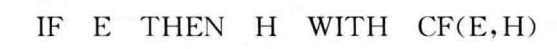## 2、专家控制

2.1 结构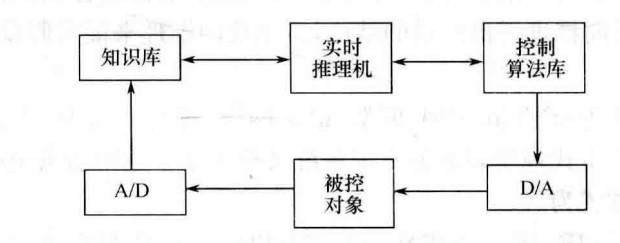## 3、专家PID控制

基于受控对象和控制规律的各种知识，无需知道被控对象的精确模型，利用专家经验来设置PID参数，专家PID控制是一种直接型专家控制器。

## 3.1 利用Python实现专家PID控制

求二阶传递函数阶跃响应的
G p ( s ) = 133 s 2 + 25 s G_p(s) = \frac{133}{s^2 + 25s}
取采样时间为1ms进行离散化。
（1）离散化，T为采样周期
s = Z − 1 − 1 T s = \frac{Z^{-1}-1}{T}
（2）代入上式得到
Y ( Z ) + 23 Y ( Z ) Z − 1 − 24 Y ( Z ) Z − 2 = 133 U ( Z ) Z − 2 Y(Z)+23Y(Z)Z^{-1}-24Y(Z)Z^{-2}=133U(Z)Z^{-2}
进一步化简得到：
Y ( Z ) = ( 2 − 25 T ) Z − 1 + ( 25 T − 1 ) Z − 2 + 133 T 2 U ( Z ) Z − 2 Y(Z)=(2-25T)Z^{-1}+(25T-1)Z^{-2}+133T^2U(Z)Z^{-2}
于是得到：
y ( k ) = ( 2 − 25 T ) y ( k − 1 ) + ( 25 T − 1 ) y ( k − 2 ) + 133 T 2 u ( k − 2 ) y(k)=(2-25T)y(k-1)+(25T-1)y(k-2)+133 T^2 u(k-2)

Python源码（该源码参考《智能控制》一书）：
主要是在程序中，构建5个专家规则库，用于限制输出。

kp = 0.7
ki = 0.02
kd = 0.1
ts = 0.001
y_1 = 0
y_2 = 0

u_1 =0
u_2 =0

size = 2000
error=[0 for i in range(size)]
y =[0 for i in range(size)]
error_1 = 0
x2_1 =0
x=[0,0,0]
times=np.linspace(0,2,size)
r = [1.0 for i in range(size)]
u = [0 for i in range(size)]
for k in range(size):
u[k] = kp*x+kd*x+ki*x
#规则1
if abs(x)>0.8:
u[k] = 0.45
elif abs(x)>0.4:
u[k] = 0.4
elif abs(x)>0.2:
u[k] = 0.12
elif abs(x)>0.01:
u[k] = 0.10

#规则2
if x*x >0 or (x==0):
if abs(x)>=0.05:
u[k] = u_1 + 2*kp*x
else:
u[k] = u_1 + 0.4*kp*x

#规则3
if (x*x<0 and x *x2_1>0) or x == 0:
u[k]=u[k]

#规则4
if x*x<0 and x *x2_1<0:
if abs(x)>=0.05:
u[k] = u_1 + 2*kp*error_1
else:
u[k] = u_1 + 0.6*kp*error_1
#规则5
if abs(x)<=0.001:
u[k] = 0.5*x+0.01*x

if u[k] >=10:
u[k] = 10

if u[k] <=-10:
u[k] = -10

y[k]=1.975*y_1 -0.975*y_2+0.000133*u_2

error[k]=r[k]-y[k]

u_2=u_1
u_1=u[k]

y_3 =y_2
y_2 =y_1
y_1 =y[k]

x = error[k]
x2_1 = x
x =(error[k]-error_1)/ts
x =x + error[k]*ts

error_1 = error[k]

plt.plot(times,y)
plt.plot(times,error)
plt.show()


运行结果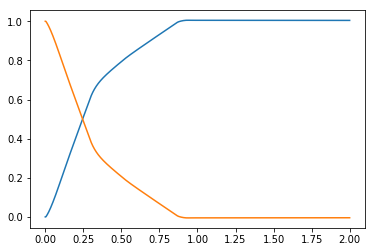展开全文• 基于Python/PyQT5的动物识别专家系统（人工智能课程实验） 本学期人工智能实验课要求完成一个动物识别专家系统。 本文采用Python+PyQT5去完成GUI的设计和程序编写。 一、系统主要逻辑： 二、系统主要代码部分...人工智能 PyQT5
• 基于Python的医疗专家推荐系统与混合推荐算法研究 基于Python的医疗专家推荐系统与混合推荐算法研究 基于Python的医疗专家推荐系统与混合推荐算法研究 基于Python的医疗专家推荐系统与混合推荐算法研究 基于Python的...
• 专家系统是一个智能计算机程序系统，其内部含有大量的某个领域专家水平的知识与经验，能够利用人类专家的知识和解决问题的方法来处理该领域问题。也就是说，专家系统是一个具有大量的专门知识与经验的程序系统，它...
• python论坛系统的设计与实现毕业论文.docx
• PYthon（SIPPY）的系统标识包 该代码的主要目的是提供不同的识别方法，以从输入-输出收集的数据开始构建动态系统的线性模型。 可以将模型构建为离散时域的传递函数或状态空间模型。 Python用户在识别算法和设置方面...
• python网络考试系统的设计与实现毕业论文.docx
• python宾馆信息管理系统设计毕业论文.docx
• 是应用人工智能技术，总结和汇集各层次各方面农业专家长期积累的宝贵经验，以及通过试验获得的各种资料和数据，总结和汇集农技推广人员和农民群众的长期实践经验，针对具体的自然条件和生态环境，用相应的计算机语言...
• 2.1运用任意一种编程语言实现动物专家识别系统并撰写实验报告 2.2功能要求： 建立一个动物识别系统的规则库，用以识别虎、豹、斑马、长颈鹿、企鹅、鸵鸟、信天翁等7种动物。为了识别这些动物，可以根据动物识别的...人工智能
• python人事信息管理系统的设计与实现毕业论文.docx
• 考察 产生式系统我猜在搜索引擎的茫茫大海中，你能看见我的这篇文章不是缘分，而是你要交作业了(抖机灵)求点赞，给作者一点分享的鼓励代码没写GUI，因为不喜欢这玩意，直接在终端中进行人机交互。使用代码之前，请...
• 原标题：给Python小白看的10个使用案例，入门Python就在这里了 今天给大家分享十个Python入门级别的小案例。这十个案例的难度不高，但是对于知识的使用非常全面，很适合小白在学习的初期建立学习信心和增加熟练度。...
• python网上书店管理系统的设计与实现毕业论文.docx
• python商品销售管理系统的设计与实现毕业论文.docx
• 人工智能(AI)在过去几年一直是前沿技术,并已逐渐进军主流应用,例如专家系统、移动设备上的个性化系统、自然语言处理领域的机器翻译、聊天机器人、无人驾驶汽车等。
• python django-备课资源推荐系统答辩.pptx
• 考察 产生式系统 代码没写GUI，因为不喜欢...不然，你在事实中输入’有奶‘，而规则库中是’有乳‘，俺这代码可不认你的’有奶‘ 文章推荐 用Python实现命题逻辑归结推理系统–人工智能 合一算法的Python实现–人工智能人工智能
• python县级城乡公共汽车车站系统的设计与实现毕业论文.docx...